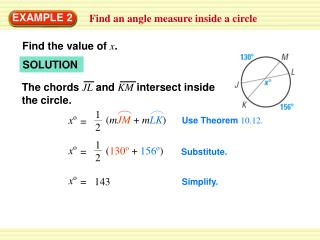DownloadDownload PresentationEXAMPLE 2

# EXAMPLE 2

Download Presentation## EXAMPLE 2

- - - - - - - - - - - - - - - - - - - - - - - - - - - E N D - - - - - - - - - - - - - - - - - - - - - - - - - - -
##### Presentation Transcript

1. The chords JLand KMintersect inside the circle. (mJM + mLK) xo = 12 12 xo (130o + 156o) = xo = 143 EXAMPLE 2 Find an angle measure inside a circle Find the value of x. SOLUTION Use Theorem 10.12. Substitute. Simplify.

2. The tangent CDand the secant CBintersect outside the circle. (mAD – mBD) m BCD = 12 12 xo (178o – 76o) = x = 51 EXAMPLE 3 Find an angle measure outside a circle Find the value of x. SOLUTION Use Theorem 10.13. Substitute. Simplify.

3. The Northern Lights are bright flashes of colored light between 50 and 200 miles above Earth. Suppose a flash occurs 150 miles above Earth. What is the measure of arc BD, the portion of Earth from which the flash is visible? (Earth’s radius is approximately 4000 miles.) EXAMPLE 4 Solve a real-world problem SCIENCE

4. Because CBand CDare tangents, Also,BC DC and CA CA . So, ABC ADC by the Hypotenuse-Leg Congruence Theorem, and BCA DCA.Solve rightCBA to find thatm BCA 74.5°. (mDEB – mBD) m BCD = CB AB and CD AD 12 12 149o [(360o – xo) –xo] xo 31 The measure of the arc from which the flash is visible is about 31o. ANSWER EXAMPLE 4 Solve a real-world problem SOLUTION Use Theorem 10.13. Substitute. Solve for x.

5. The chords ACand CDintersect inside the circle. = 12 12 78o (yo + 95o) = 61 = y for Examples 2, 3, and 4 GUIDED PRACTICE 4. Find the value of the variable. SOLUTION (mAB + mCD) 78° Use Theorem 10.12. Substitute. Simplify.

6. SOLUTION The tangent JFand the secant JGintersect outside the circle. (mFG – mKH) m FJG = 12 12 30o (ao – 44o) = a = 104 for Examples 2, 3, and 4 GUIDED PRACTICE Find the value of the variable. 5. Use Theorem 10.13. Substitute. Simplify.

7. Because QTand QRare tangents, Also,TS SR and CA CA . So, QTS QRS by the Hypotenuse-Leg Congruence Theorem, and (mTUR – mTR) m TQR QR RS and QT TS 12 12 TQS RQS.Solve rightQTS to find thatm RQS 73.7°. = 73.7o [(xo) –(360 –x)o] xo 253.7 for Examples 2, 3, and 4 GUIDED PRACTICE 6. Find the value of the variable. SOLUTION Use Theorem 10.13. Substitute. Solve for x.